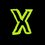# Colouring proofs for beginners

Can anybody please explain me with examples, how to solve tough colouring related problems ( as in CRMO-4 question paper).

Please give me some advice on how to solve these kind of problems.

PS: I've already tried Arthur Engel. So please give me important methods and not the basic idea.Note by Priyansh Sangule
6 years, 7 months ago

This discussion board is a place to discuss our Daily Challenges and the math and science related to those challenges. Explanations are more than just a solution — they should explain the steps and thinking strategies that you used to obtain the solution. Comments should further the discussion of math and science.

When posting on Brilliant:

• Use the emojis to react to an explanation, whether you're congratulating a job well done , or just really confused .
• Ask specific questions about the challenge or the steps in somebody's explanation. Well-posed questions can add a lot to the discussion, but posting "I don't understand!" doesn't help anyone.
• Try to contribute something new to the discussion, whether it is an extension, generalization or other idea related to the challenge.

MarkdownAppears as
*italics* or _italics_ italics
**bold** or __bold__ bold
- bulleted- list
• bulleted
• list
1. numbered2. list
1. numbered
2. list
Note: you must add a full line of space before and after lists for them to show up correctly
paragraph 1paragraph 2

paragraph 1

paragraph 2

[example link](https://brilliant.org)example link
> This is a quote
This is a quote
    # I indented these lines
# 4 spaces, and now they show
# up as a code block.

print "hello world"
# I indented these lines
# 4 spaces, and now they show
# up as a code block.

print "hello world"
MathAppears as
Remember to wrap math in $$ ... $$ or $ ... $ to ensure proper formatting.
2 \times 3 $2 \times 3$
2^{34} $2^{34}$
a_{i-1} $a_{i-1}$
\frac{2}{3} $\frac{2}{3}$
\sqrt{2} $\sqrt{2}$
\sum_{i=1}^3 $\sum_{i=1}^3$
\sin \theta $\sin \theta$
\boxed{123} $\boxed{123}$

Sort by:

here's the answer- Since there are three red vertices than among the remaining 17 vertices there are nine of them of the same colour, say blue. We can divide the vertices of the regular 20-gon into four disjoint sets such that each set consists of vertices that form a regular pentagon. Since there are nine blue points, at least one of these sets will have three blue points. Since any three points on a pentagon form an isosceles triangle, the statement follows

another solution-

divide the 20-gon in 4 pentagon so that we can have isosceles triangle in each of these pentagon by joining any three vertices. now as there are 3 red vertices than we are left with 17 vertices. now there will be 1 pentagon which will not have any red vertice as by pigeonhole principle and also by pigeonhole principle that pentagon should have 3 vertices with same colour. hence it follows that there are 3 vertices with same colour such that they form an isosceles triangle.

and btw priyansh did u qualified RMO?

- 6 years, 7 months ago

No Aditya, I couldn't but some of my friends did. And Thanx for the solution !

- 6 years, 6 months ago

Hi priyansh sangule, I suggest that you wait for a while because I will soon talk about imo standard coloring problens in my daily elementary techniques in the imo posts.

- 6 years, 7 months ago

Thank you!

- 6 years, 7 months ago